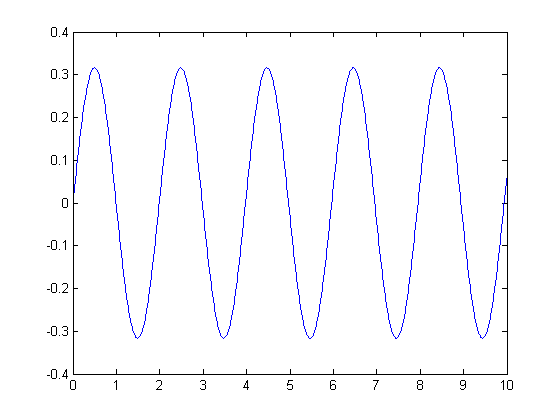# ODEs, from Symbolic to Numeric Code

In a recent post on solving ODEs, the reader Jason wondered if there was a way to do away with the manual algebraic steps using Symbolic Math Toolbox. There currently isn't a canned way to do it so the result is a MATLAB program, but you can do so in MuPAD, the symbolic engine for Symbolic Math Toolbox. For now, I want to show you how you might generate the ODE solution in the case where MuPAD finds a closed-form solution.

### Define Symbolic Variables

Let's first define the variables we need to describe the equations for a simple harmonic oscillator, using the same notation as the post linked above.

syms x t m k

### Solve the System

Using the function dsolve, we find the solution.

sol = dsolve('m*D2x+k*x(t)','x(0) = 0','Dx(0) = 1','t');

Reformat the solution display suitable for posting.

csol = char(sol);
disp(csol(1:45))
disp(csol(46:end))
(m*exp((t*(-k*m)^(1/2))/m))/(2*(-k*m)^(1/2))
- m/(2*exp((t*(-k*m)^(1/2))/m)*(-k*m)^(1/2))


### Convert Solution from MuPAD

Using matlabFunction, I next generate a function handle to a MATLAB version of the solution.

f = matlabFunction(sol);

Again, reformat expression to display more nicely in post.

cf = func2str(f);
disp(cf(1:65))
disp(['       ', cf(66:end)])
@(k,m,t)m.*exp((t.*sqrt(-k.*m))./m).*1.0./sqrt(-k.*m).*(1.0./2.0)
-m.*exp(-(t.*sqrt(-k.*m))./m).*1.0./sqrt(-k.*m).*(1.0./2.0)


### Set Values for Mass and Spring Constant

I'm using the same values for k and m that I used in the referenced post.

k = 10;
m = 1;

I'm now creating a short-hand function with the values for k and m set so I can plot the result.

fnew = @(t) f(k,m,t);

### Plot the Solution

t = 0:0.01:10;
plot(t,fnew(t))### All Well and Good IF ...

That's all well and good if MuPAD can find an analytic solution to the differential equation. For now, there is not a way to automatically generate a MATLAB program for the system of equations defining the ODE suitable for solving numerically. MuPAD itself has numerical ODE solvers which may be suitable. Do you have problems that straddle the symbolic and numerical worlds? How do you handle them? Let me know here.

Published with MATLAB® 7.10

|

### Comments

To leave a comment, please click here to sign in to your MathWorks Account or create a new one.